# Molar Concentration Knowing the concentration of a solution

• Slides: 13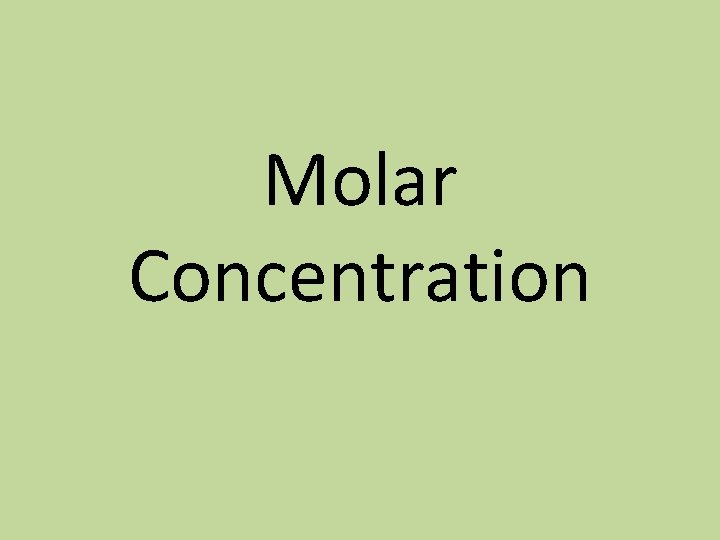Molar Concentration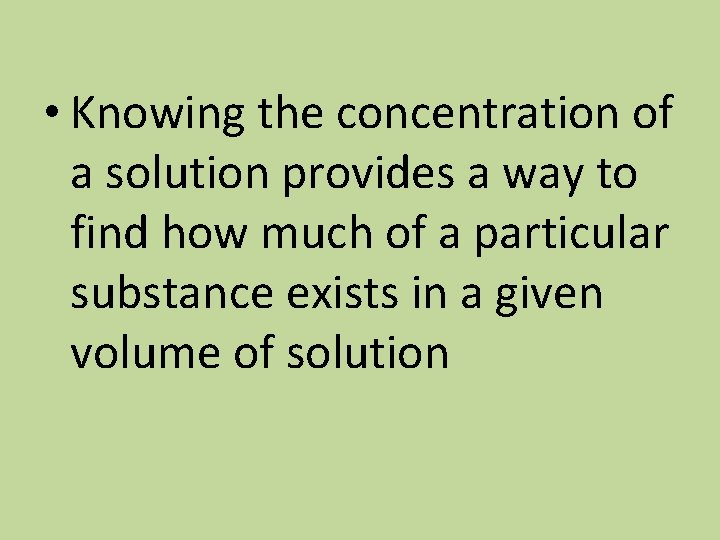• Knowing the concentration of a solution provides a way to find how much of a particular substance exists in a given volume of solutionConcentration • The amount of a specific substance in a known volume of solution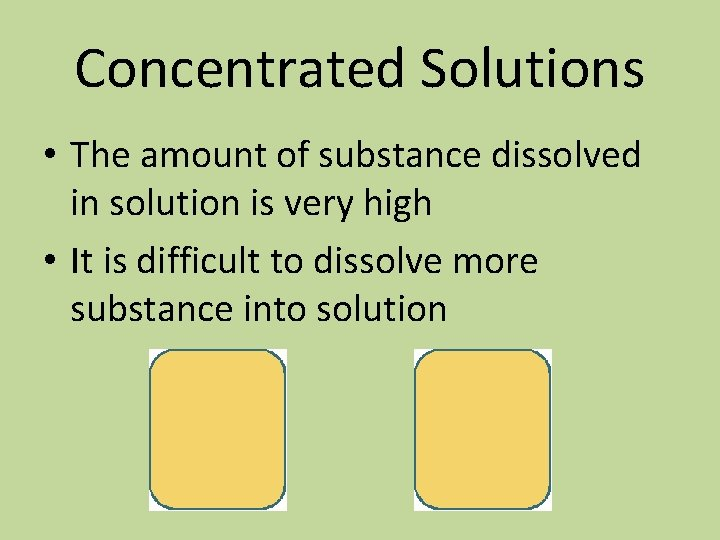Concentrated Solutions • The amount of substance dissolved in solution is very high • It is difficult to dissolve more substance into solutionDilute Solutions • The solution has a relatively low concentration • Very little substance dissolved in the solution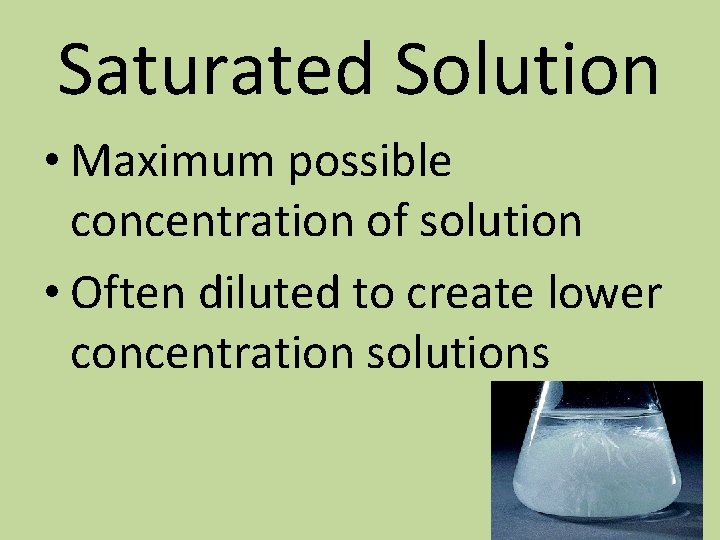Saturated Solution • Maximum possible concentration of solution • Often diluted to create lower concentration solutionsSupersaturates solution • Heated up to increase solute solubility and then carefully cooled! • i. e. Jello!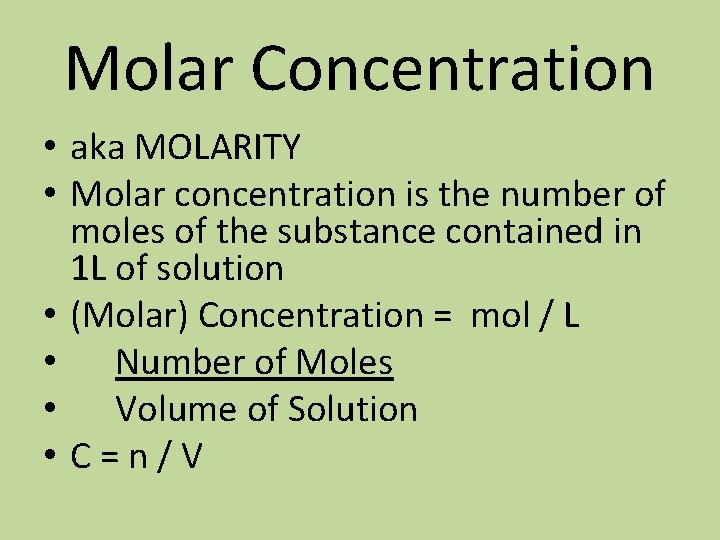Molar Concentration • aka MOLARITY • Molar concentration is the number of moles of the substance contained in 1 L of solution • (Molar) Concentration = mol / L • Number of Moles • Volume of Solution • C=n/V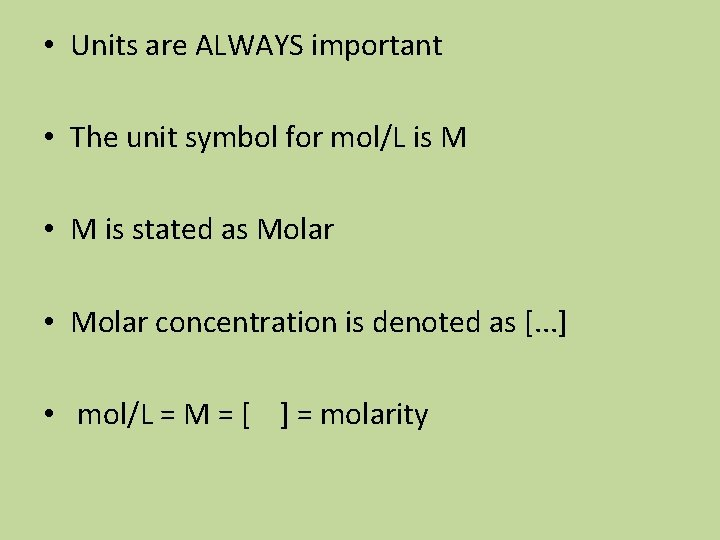• Units are ALWAYS important • The unit symbol for mol/L is M • M is stated as Molar • Molar concentration is denoted as [. . . ] • mol/L = M = [ ] = molarity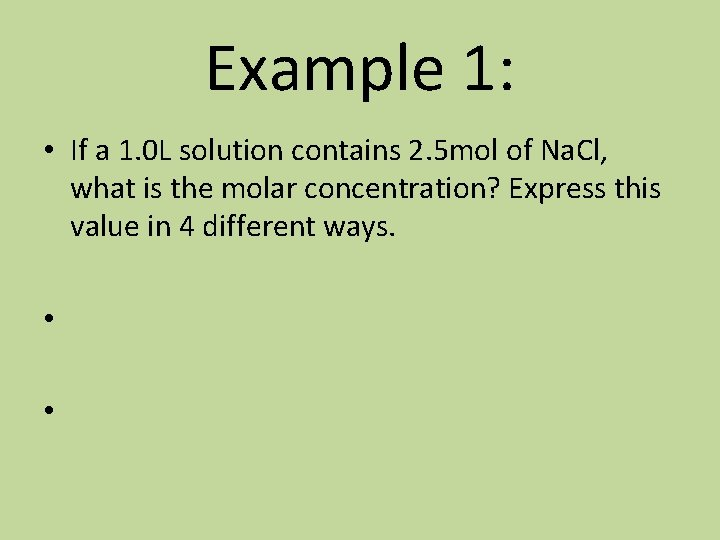Example 1: • If a 1. 0 L solution contains 2. 5 mol of Na. Cl, what is the molar concentration? Express this value in 4 different ways. • •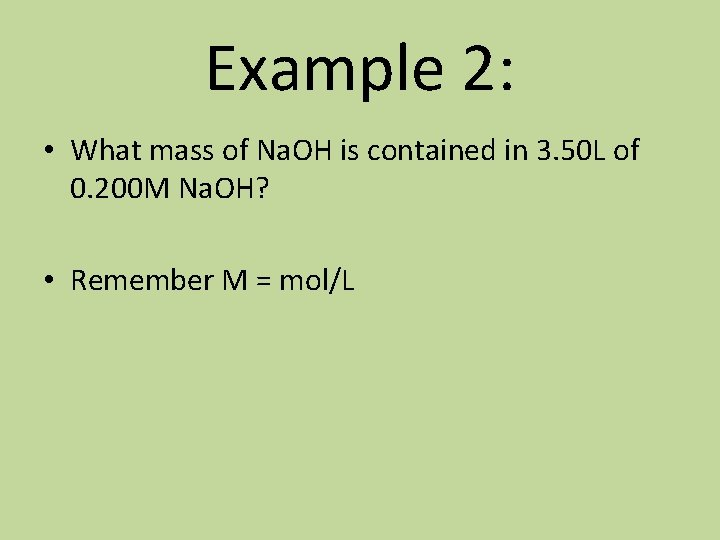Example 2: • What mass of Na. OH is contained in 3. 50 L of 0. 200 M Na. OH? • Remember M = mol/L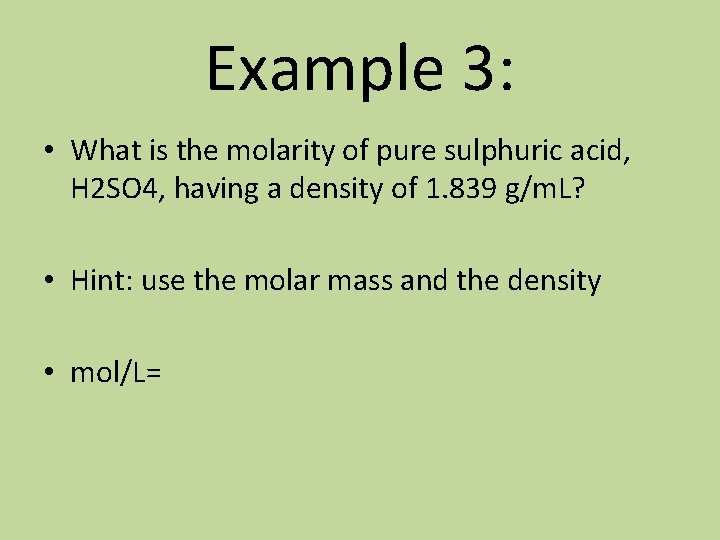Example 3: • What is the molarity of pure sulphuric acid, H 2 SO 4, having a density of 1. 839 g/m. L? • Hint: use the molar mass and the density • mol/L=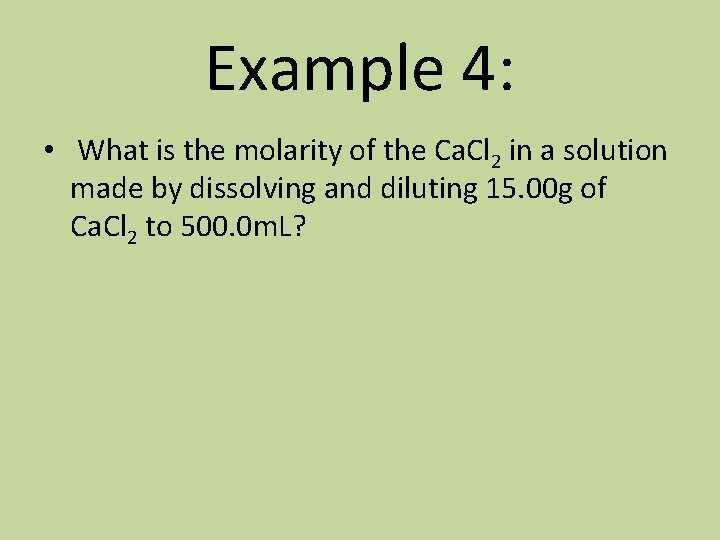Example 4: • What is the molarity of the Ca. Cl 2 in a solution made by dissolving and diluting 15. 00 g of Ca. Cl 2 to 500. 0 m. L?